﻿ Two Phase Horizontal Separators Sizing | Oil & Gas Process Engineering

## Two Phase Horizontal Separators Sizing

For sizing a horizontal separator it is necessary to choose a seam-toseam  vessel length and a diameter. This choice must satisfy the conditions  for gas capacity that allow the liquid drops to fall from the gas to the liquid volume as the gas traverses the effective length of the vessel. It must also  provide sufficient retention time to allow the liquid to reach equilibrium.

For a vessel 50% full of liquid, and separation of 100-micron liquid  drops from the gas, the following equations apply:

Gas Capacity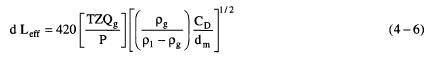where d= vessel internal diameter, in.
Leff= effective length of the vessel where separation occurs, ft
T = operating temperature, °R
Qg= gas flow rate, MMscfd
P= operating pressure, psia
Z= gas compressibility
CD= drag coefficient
dm= liquid drop to be separated, micron
pg= density of gas, lb/ft3
p1 = density of liquid, lb/ft3

Derivation of Equation 4-6
Assume vessel is one-half full of liquid. Determine gas velocity, Vg. A  is in ft2, D in ft, d in inches, Q in ft3/s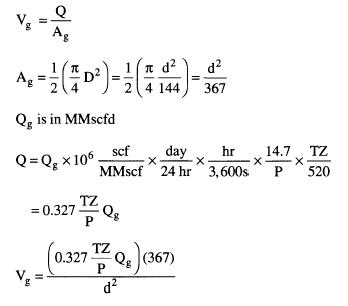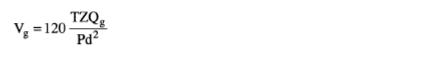Set the residence time of the gas equal to the time required for the droplet to fall to the gas-liquid interface: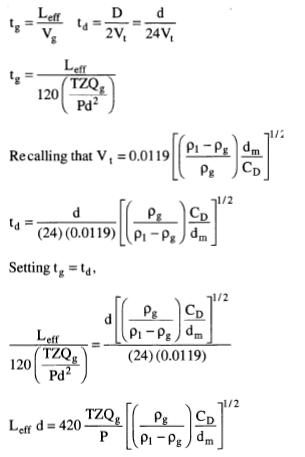Liquid Capacitywhere t,. = desired retention time for the liquid, min
Q1 = liquid flow rate, bpd
Derivation of Equation 4-7
t is in s, Vol in ft3, Q in ft3/s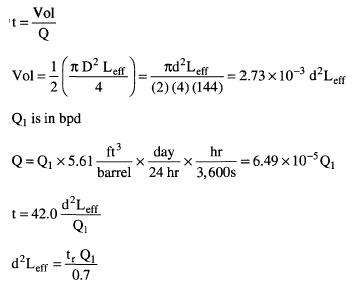Seam-to-Seam Length and Slenderness Ratio
The seam-to-seam length of the vessel should be determined from the  geometry once an effective length has been determined. Allowance must  be made for the inlet diverter and mist extractor. For screening purposes  the following approximation has been proven useful: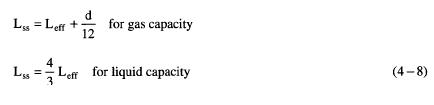Equations 4-6 and 4-7 allow for various choices of diameter and  length. It can be shown that the smaller the diameter the less the vessel  will weigh and thus the lower its cost. There is a point, however, where
decreasing the diameter increases the possibility that high velocity in the  gas flow will create waves and re-entrain liquids at the gas-liquid interface.  Experience has shown that if the gas capacity governs and the length divided by the diameter (slenderness ratio) is greater than 4 or 5,  re-entrainment could become a problem. Equation 4-8 indicates that slenderness  ratios must be at least 1 or more. Most common separators are  designed for slenderness ratios of 3 to 4.

Procedure for Sizing Horizontal Separators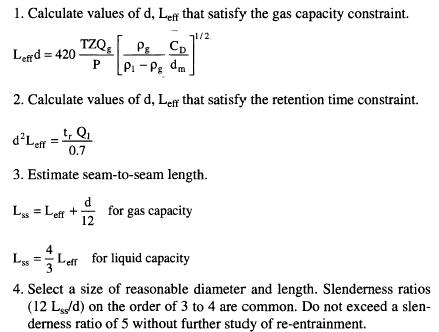For separators other than 50% full of liquid, equations can be derived  similarly, using the actual gas and liquid areas to calculate gas velocity and liquid volume. The equations are derived using the same principles.